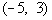Krishna
0

Step 1: Write the quadratic inequality in standard form.

EXAMPLE: x^2\ +\ 2x\ <15

x^2\ +\ 2x\ -15\ <0

Step 2: Solve the quadratic equation ax^2+bx+c, by factoring to get the boundary point(s).

EXAMPLE: x^2 + 2x - 15 = 0

(x+5)(x-3) = 0

x = -5 or x = 3

Step 3: Use the boundary points found in step 2 to mark off test intervals on the number line and list all of the factors found in step 2.

EXAMPLE: Note that the two boundary points create three sections (-\infty, -5), (-5, 3), (3, \infty),

Step 4 : Substitute the numbers in the intervals on the quadratic equation, and make sure is it satisfying the inequality or not

EXAMPLE: If we chose a number in the first interval, (-\infty, -5), like -6 (I could have used -10, -25, or -10000 as long as it is in the interval), it would make both factors negative:

x + 5 = 0    and  x - 3 =0

-6 + 5 = -1 and -6 - 3 = -9

(-1)(-9) < 0

9 <  0 not satisfied,

take the another interval and verify

If we chose a number in the second interval,, like 0 (I could have used -4, -1, or 2 as long as it is in the interval), it would make x + 5 positive and x - 3 negative:

0 + 5 = 5 and 0 - 3 = -3

(5)(-3) < 0

-8 < 0 satisfying

Step 5: Take the interval which satisfies the inequality and find out the suitable Interval notation

NOTE: Open interval indicating all values between -5 and 3.

Closed intervals indicating all values less than or equal to -5 or greater than or equal to 3﻿